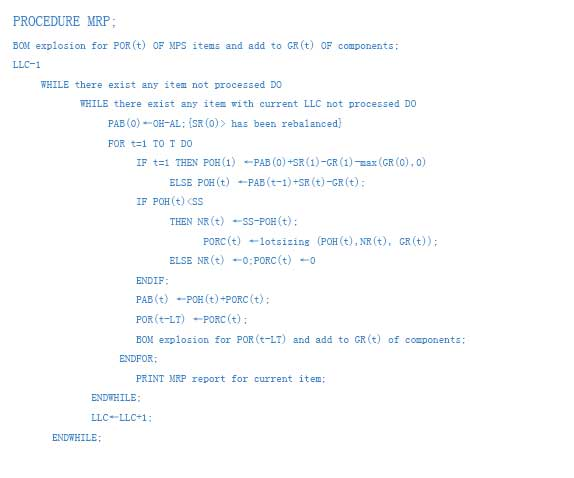﻿ suzhouHDD.com苏州硬盘数据恢复中心_ERP算法原理_吴江数据恢复|常熟数据恢复|昆山数据恢复|张家港数据恢复

• 孝悌忠信
• 立身之本
• 礼义廉耻
• 吾辈所宗
• 万古纲常
• 千秋名节

### 客户服务### HDD相关知识## ERP核心算法

GR(t)=Co(t),for 0<=t<=DTF;

GR(t)=MAX(CO(t),FCS(t)), for DTF

GR(t)=FCS(t), for PTF

POH(t)=PAB(t-1)+SR(t)-GR(t), for 2<=t<=T

L4L PORC(t)=NR(t),for 1<=t<=T

PAB(t)=POH(t)+PORC(t), FOR 1<=t<=T

MPS（t）=SR（t）+PORC(t), FOR 1<=t<=T. PORC 是系统建议补充但尚未发单的料，SR则是已发出定单的料。NR经LSR及ST 调整后就成为PORC，这个量发出后是SR；PORC 向前推移一个LT即得POR；

父件的POR会被BOM展开，算出其所有子件的GR；当计划定单发出时，POR就变成SR，POR就不再存在了；

LSR （lot size rule）订货策略

LS (Lot size) 批量

OH(on –hand)现有库存量

SS(safety Stock)安全库存量

ST(Safety Time)安全时间

ST是使PORC及POR 同时提前的一个时间值;供应商迟交的原因不是LT短，而是态度或管理有问题;ST是解决提前期综合症的Solution;

AL(Allocated)已分配量/保留量 预定从仓库领出但实际尚未领出的数量

LLC（Low level Code）低层代码/低阶代码

MRP算法的实质就是有别于ROP(Re-order Point)的TPOP（Time Phased Order Point),核心就是NR=GR-INV!